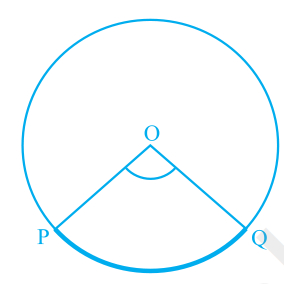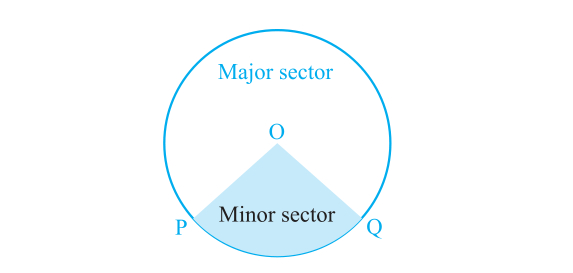Angle at the centre of a circle by an arc

## Objective:

To verify that the angle subtended by an arc at the centre of a circle is twice the angle subtended by the same arc at any other point on the remaining part of the circle.### Circles

• A circle is a set of points which are all a certain distance from a fixed point known as the centre.
• A line joining the centre of a circle to any of the points on the circle is known as a radius.
• The circumference of a circle is the length of the circle. The circumference of a circle = 2 × π × the radius.## Terminology

The following is a recap of terms that are regularly used when referring to circles.

Arc: An arc is a part of the circumference of a circle.

Chord: A chord is a straight line joining the ends of an arc.

Radius: A radius, r, is any straight line from the centre of the circle to a point on the circumference.

Segment: A segment is the part of the circle that is cut off by a chord. A chord divides a circle into two segments.

Angle Subtended: In geometry, an angle subtended by an arc, line, or other curve is one whose two rays pass through the endpoints of the arc.